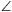Mathematical and Physical Journal
for High Schools
Issued by the MATFUND Foundation
 Already signed up? New to KöMaL?

# KöMaL Problems in Mathematics, April 2012

Please read the rules of the competition.

Show/hide problems of signs:## Problems with sign 'C'

Deadline expired on May 10, 2012.

C. 1120. Represent those points P(x;y) on the coordinate plane whose coordinates satisfy |y|1-x and |x|3-y.

(5 pont)

solution (in Hungarian), statistics

C. 1121. Prove that if n is a natural number then the value of the sumis an integer.

(5 pont)

solution (in Hungarian), statistics

C. 1122. The angle bisector of angle BAC of a triangle ABC intersects side BC at A1. Let the centres of the circumscribed circles of triangles ABC, ABA1, ACA1 be O, O1, O2, respectively. Prove that triangle OO1O2 is isosceles.

(5 pont)

solution (in Hungarian), statistics

C. 1123. A 4×4×4 cube is built out of sugar cubes. How many different cuboids (that differ in at least one sugar cube) are formed by the sugar cubes?

(5 pont)

solution (in Hungarian), statistics

C. 1124. Solve the following simultaneous equations: xx+y=y3, yx+y=x12.

(5 pont)

solution (in Hungarian), statistics## Problems with sign 'B'

Deadline expired on May 10, 2012.

B. 4442. Ann, Bill and Cecilia play the following game. They take turns choosing an integer between 1 and 10, and adding that number to the sum of the numbers that have been said so far. (For simplicity, in each turn, the player states the sum.) The player who is first able to say 100 wins the game. Prove that the two girls, with the appropriate strategy, are able to achieve that one of them is the winner.

Suggested by B. Futó, New York

(3 pont)

solution (in Hungarian), statistics

B. 4443. a1,a2,...,an és b1,b2,...,bk are two given sequences with positive integer terms with aik and bjn. Show that there are integers 0i1<i2n and 0j1<j2k such that.

(5 pont)

solution (in Hungarian), statistics

B. 4444. The points E and G, respectively, are obtained by rotating vertex D of a square ABCD about A and vertex B (lying opposite D) about C outwards, through the same acute angle. The intersection of lines AE and BG is F. Line GD intersects the circles GCB and BDE again at points L and M, respectively. Prove that the points A, B, F, L, M are cyclic.

Suggested by J. Bodnár

(3 pont)

solution (in Hungarian), statistics

B. 4445. A convex solid has six square faces and eight regular hexagonal faces. Given that no two square faces have a vertex in common, and the solid has an edge of unit length, determine the volume of the solid.

(4 pont)

solution (in Hungarian), statistics

B. 4446. How many squares are formed by the lattice points in an n×n square lattice?

(4 pont)

solution (in Hungarian), statistics

B. 4447. Solve the equation.

(4 pont)

solution (in Hungarian), statistics

B. 4448. The escribed circle drawn to side AC of a triangle ABC touches the lines of sides BC, AC and AB at the points A1, B1 and C1, respectively. Let F denote the midpoint of the line segment A1B1. Prove thatB1C1C=A1C1F.

(4 pont)

solution (in Hungarian), statistics

B. 4449. In how many zeros does the number 456+654 end in decimal notation?

(4 pont)

solution (in Hungarian), statistics

B. 4450. AB and C are three distinct given points lying on the line e in this order. What is the locus of the points P for which the inscribed circle of triangle ACP touches line e at B?

(4 pont)

solution (in Hungarian), statistics

B. 4451. Consider a convex n-sided polygon that has no four vertices lying on the same circle. Three vertices are selected in every possible way, and the circle passing through the three points is drawn. Such a circle is called thin if the vertices of the polygon (except the vertices of the triangle) all lie outside the circle. The circle is called fat if all vertices of the polygon lie on the closed disc bounded by the circle. Are there more thin circles or more fat circles?

Suggested by S. Róka, Nyíregyháza

(5 pont)

solution (in Hungarian), statistics## Problems with sign 'A'

Deadline expired on May 10, 2012.

A. 560. Given a right circular cone with vertex O, and a fixed point P in the base plane of the cone. Draw a line x through P which intersects the base circle of the cone at two points, X1 and X2. Show thatdoes not depend on the choice of the line x.

(5 pont)

solution, statistics

A. 561. Show thatholds for all positive numbers a, b, cp.

(5 pont)

solution, statistics

A. 562. Let f(k)=2k+1 for arbitrary positive integer k. Is there any positive integer n which divides f(f(n)), but does not divide f(f(f(n)))?

(5 pont)

statistics

### Upload your solutions above or send them to the following address:

KöMaL Szerkesztőség (KöMaL feladatok),
Budapest 112, Pf. 32. 1518, Hungary